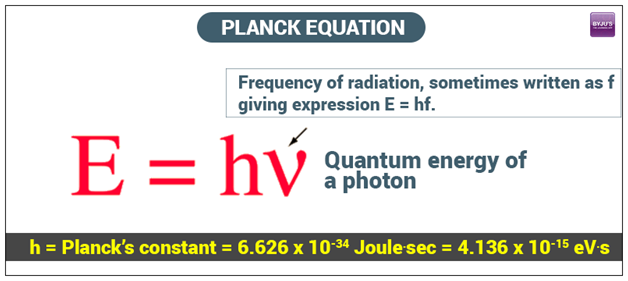# Planck's Equation### What is Planck’s Equation?

Max Planck discovered a theory that energy is transferred in the form of chunks called quanta, assigned as h. The variable h holds the constant value of 6.63 x 10-34 J.s based on the International System of Units, and the variable describes the frequency in s-1. Planck’s law helps us calculate the energy of photons when their frequency is known.

If the wavelength is known, you can calculate the energy using the wave equation to calculate the frequency and then apply Planck’s equation to find the energy.

### What is Planck’s Constant?

Put differently, Plank’s constant describes the relevancy between the energy per quantum (photon) of electromagnetic radiation and its frequency.

#### Solved Example:

Green light has a wavelength of 525 nm. Determine the energy for the green light in joules.

#### Solution:

To find the Frequency;

As we know that,

$$\begin{array}{l}c=\lambda \times v\end{array}$$

$$\begin{array}{l}v=\frac{3 \times 10^{8}}{525}\end{array}$$

Hence,

$$\begin{array}{l}v = 5.71 \times 10^{14}/s\end{array}$$

To find the Energy;

As we know that

$$\begin{array}{l}E = h \times \nu\end{array}$$

$$\begin{array}{l}(6.626 \times 10^{-34 })\times (5.71 \times 10^{14})\end{array}$$

$$\begin{array}{l}3.78 \times 10^{-19} J/photon\end{array}$$

### Planck’s Law:

It states that electromagnetic radiation from heated bodies is not emitted as a continuous flow but is made up of discrete units or quanta of energy, the size of which involves a fundamental physical constant (Planck’s constant).

Mathematically,

$$\begin{array}{l}B_{\lambda }(T)=\frac{2hc^{2}}{\lambda ^{5}}\frac{1}{e^{\frac{hc}{kT\lambda }}-1}\end{array}$$

Where,

h = Planck’s Constant

$$\begin{array}{l}=6.62\times 10^{-34}Js\end{array}$$

k = Boltzmann’s Constant = 1.381 × 10-23 J/K

## Frequently Asked Questions – FAQs

Q1

### What is the significance of Planck’s equation?

Using Planck’s equation, we can connect the relationship between spectral emissivity, temperature, and radiant energy.
Q2

### What is the unit of Planck’s Constant?

Planck’s equation unit = Joule – sec.
Q3

### What is the Planck constant?

6.626176 x 10-34 joule-seconds.
Q4

[ML2T−1]
Q5

### Which of the following scientific quantities has the same dimensional formula as Planck’s constant?

Angular momentum

Stay tuned to BYJU’S to know more about Planck’s law and Planck’s quantum theory along with worked problems and solutions.

Test your Knowledge on Planck Equation